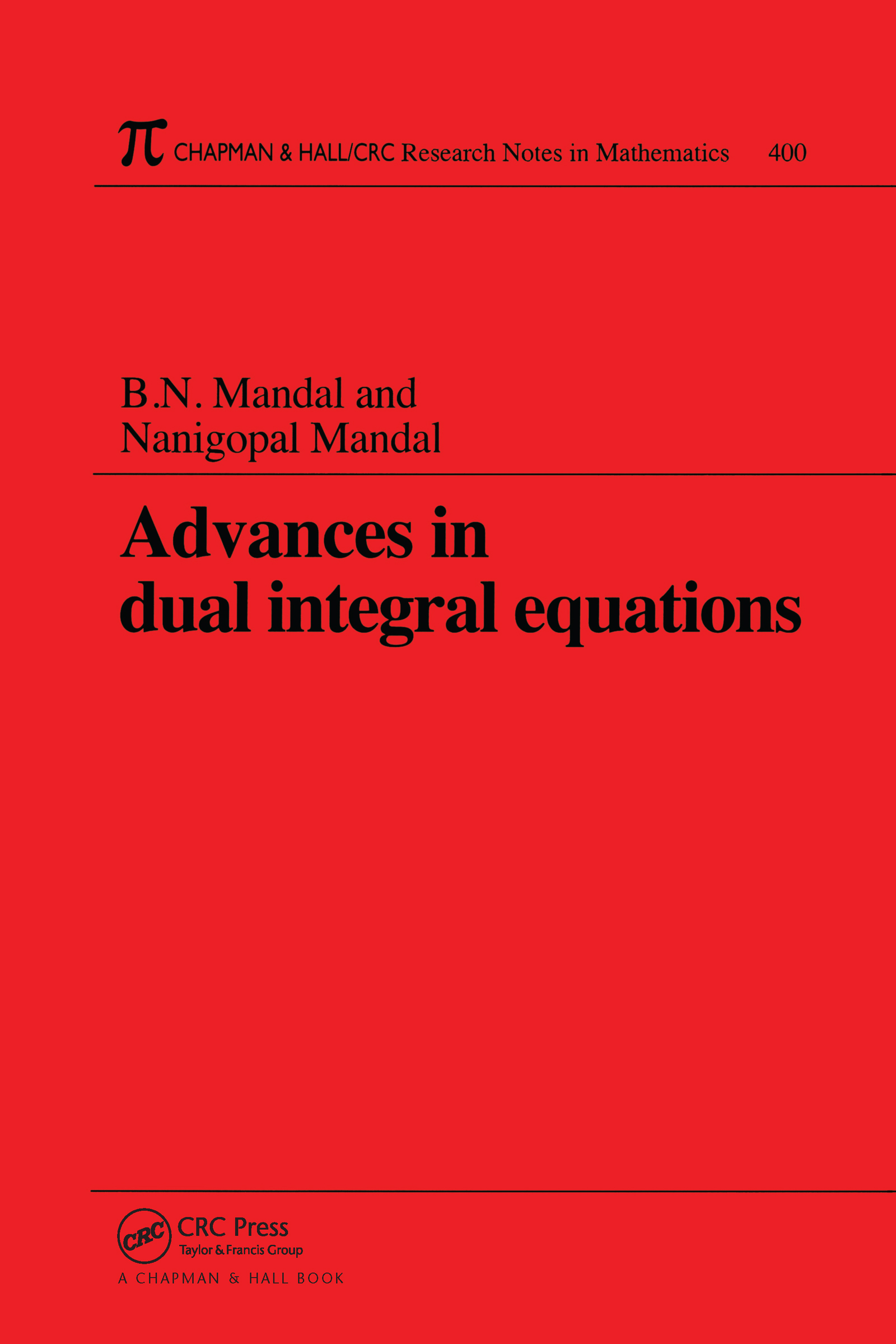Advances in Dual Integral Equations

1st Edition

Chapman and Hall/CRC

232 pages

Purchasing Options:\$ = USD
Paperback: 9780849306174
pub: 1998-12-18
SAVE ~\$35.00
Currently out of stock
\$175.00
\$140.00
x

FREE Standard Shipping!

Description

The effectiveness of dual integral equations for handling mixed boundary value problems has established them as an important tool for applied mathematicians. Their many applications in mathematical physics have prompted extensive research over the last 25 years, and many researchers have made significant contributions to the methodology of solving and to the applications of dual integral equations. However, until now, much of this work has been available only in the form of research papers scattered throughout different journals.

In Advances in Dual Integral Equations, the authors systematically present some of the recent developments in dual integral equations involving various special functions as kernel. They examine dual integral equations with Bessel, Legendre, and trigonometric functions as kernel plus dual integral equations involving inverse Mellin transforms. These can be particularly useful in studying certain mixed boundary value problems involving homogeneous media in continuum mechanics. However, when dealing with problems involving non-homogenous media, the corresponding equations may have different kernels. This application prompts the authors to conclude with a discussion of hybrid dual integral equations-mixed kernels with generalized associated Legendre functions and mixed kernels involving Bessel functions.

Researchers in the theory of elasticity, fluid dynamics, and mathematical physics will find Advances in Dual Integral Equations a concise, one-stop resource for recent work addressing special functions as kernel.

Introduction

An Overview of Dual Integral Equations

Two Special Methods for Solving Some Classes of Dual Integral Equations

Dual Integral Equations with Bessel Function Kernel

Kernels Involving a Bessel Function of the First Kind

Kernels Involving a Bessel Function of the Second Kind

Dual Integral Equations Related to the Kontorovich-Levedev Transform

Dual Integral Equations Associated with Inverse Weber-Orr Transforms

Dual Integral Equations with Spherical Harmonic Kernel

Kernels Involving Legendre Functions

Kernels Involving Associated Legendre Functions

Kernels Involving Generalized Associated Legendre Functions

Dual Integral Equations with Trigonometric Function Kernel

Some Elementary Methods

Solutions by Using the Generalized Mehler-Fock Inversion Theorem

Solutions by Using the Generalized Associated Mehler-Fock Inversion Theorem

Dual Integral Equations Involving Inverse Mellin Transforms

Hybrid Dual Integral Equations

Mixed Kernels with Generalized Associated Legendre Functions

Mixed Kernels Involving Bessel Functions

Appendix: Useful Results of some Special Functions

Bessel Functions

Legendre and Associated Legendre Functions

Generalized Associated Legendre Functions

Bibliography

Index